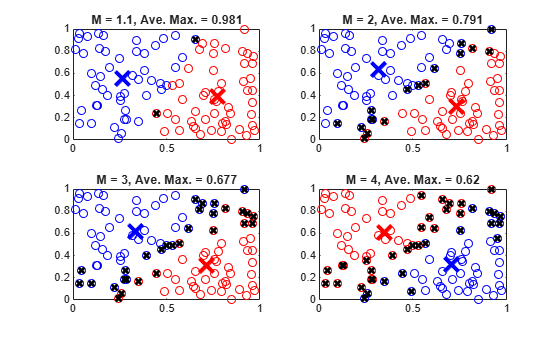# Adjust Fuzzy Overlap in Fuzzy C-Means Clustering

This example shows how to adjust the amount of fuzzy overlap when performing fuzzy c-means clustering.

Create a random data set. For reproducibility, initialize the random number generator to its default value.

```rng("default") data = rand(100,2);```

Specify fuzzy partition matrix exponents.

`M = [1.1 2.0 3.0 4.0];`

The exponent values in `M` must be greater than `1`, with smaller values specifying a lower degree of fuzzy overlap. In other words, as `M` approaches `1`, the boundaries between the clusters become more crisp.

For each overlap exponent:

1. Cluster the data.

2. Classify each data point into the cluster for which it has the highest degree of membership.

3. Find the data points with maximum membership values below `0.6`. These points have a more fuzzy classification.

4. To quantify the degree of fuzzy overlap, calculate the average maximum membership value across all data points. A higher average maximum membership value indicates that there is less fuzzy overlap.

5. Plot the clustering results.

```for i = 1:4 % 1. Cluster the data. options = fcmOptions(... NumClusters=2,... Exponent=M(i),... Verbose=false); [centers,U] = fcm(data,options); % 2. Classify the data points. maxU = max(U); index1 = find(U(1,:) == maxU); index2 = find(U(2,:) == maxU); % 3. Find data points with lower maximum membership values. index3 = find(maxU < 0.6); % 4. Calculate the average maximum membership value. averageMax = mean(maxU); % 5. Plot the results. subplot(2,2,i) plot(data(index1,1),data(index1,2),"ob") hold on plot(data(index2,1),data(index2,2),"or") plot(data(index3,1),data(index3,2),"xk",... LineWidth=2) plot(centers(1,1),centers(1,2),"xb",... MarkerSize=15,LineWidth=3) plot(centers(2,1),centers(2,2),"xr",... MarkerSize=15,LineWidth=3) hold off title("M = " + num2str(M(i)) + ... ", Ave. Max. = " + num2str(averageMax,3)) end```A given data point is classified into the cluster for which it has the highest membership value, as indicated by `maxU`. A maximum membership value of `0.5` indicates that the point belongs to both clusters equally. The data points marked with a black x have maximum membership values below `0.6`. These points have a greater degree of uncertainty in their cluster membership.

More data points with low maximum membership values indicate a greater degree of fuzzy overlap in the clustering result. The average maximum membership value, `averageMax`, provides a quantitative description of the overlap. An `averageMax` value of `1` indicates crisp clusters, with smaller values indicating more overlap.